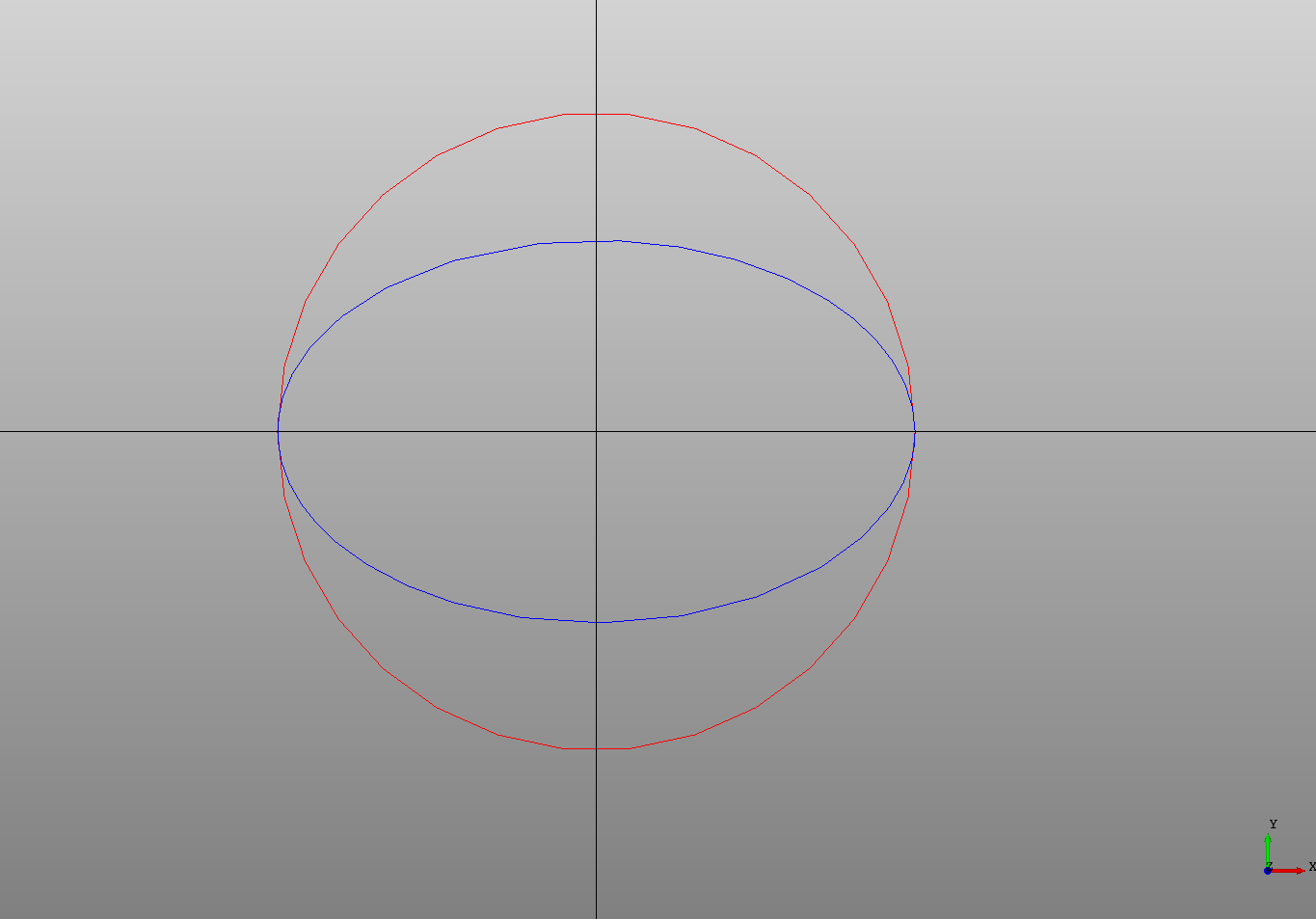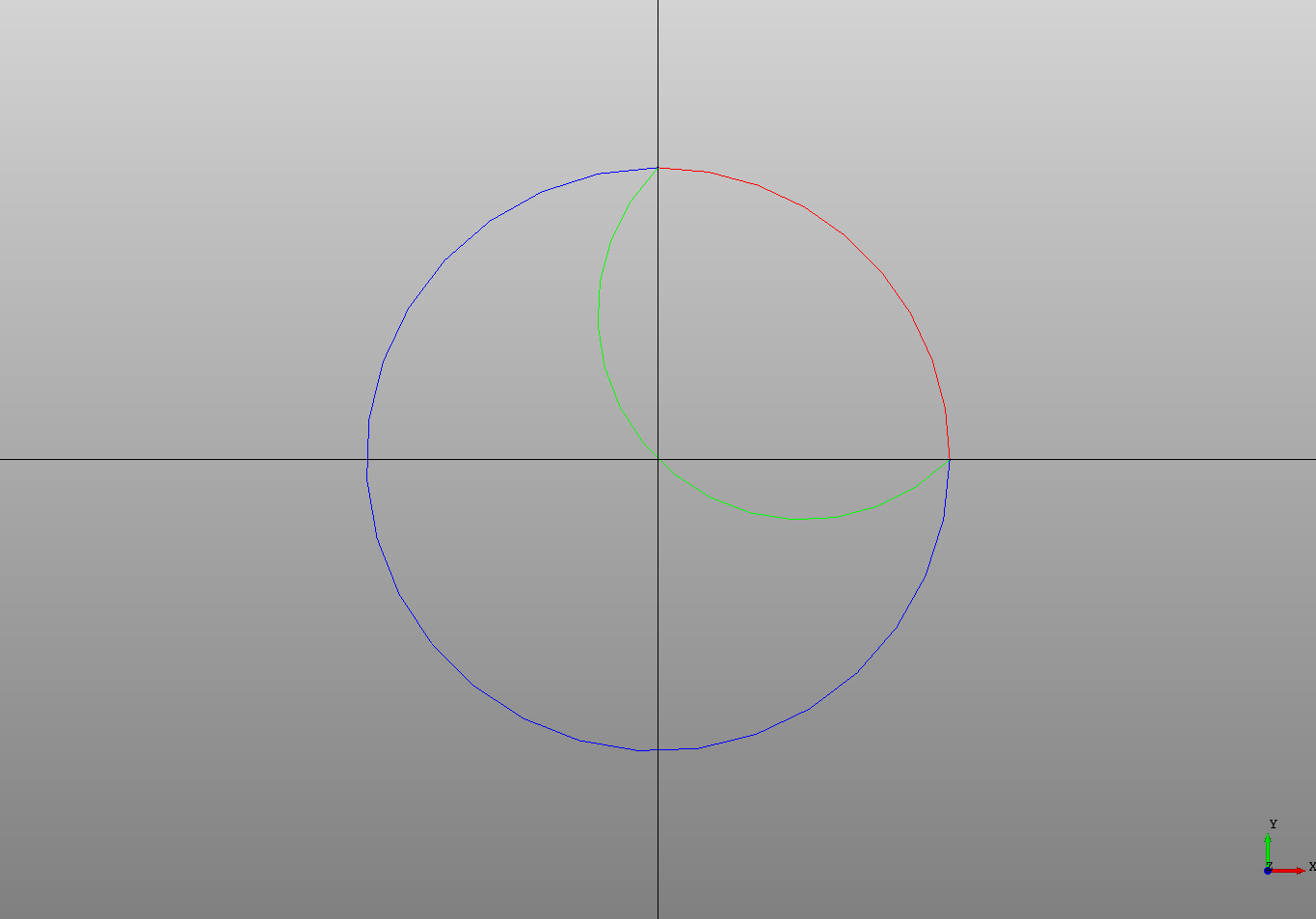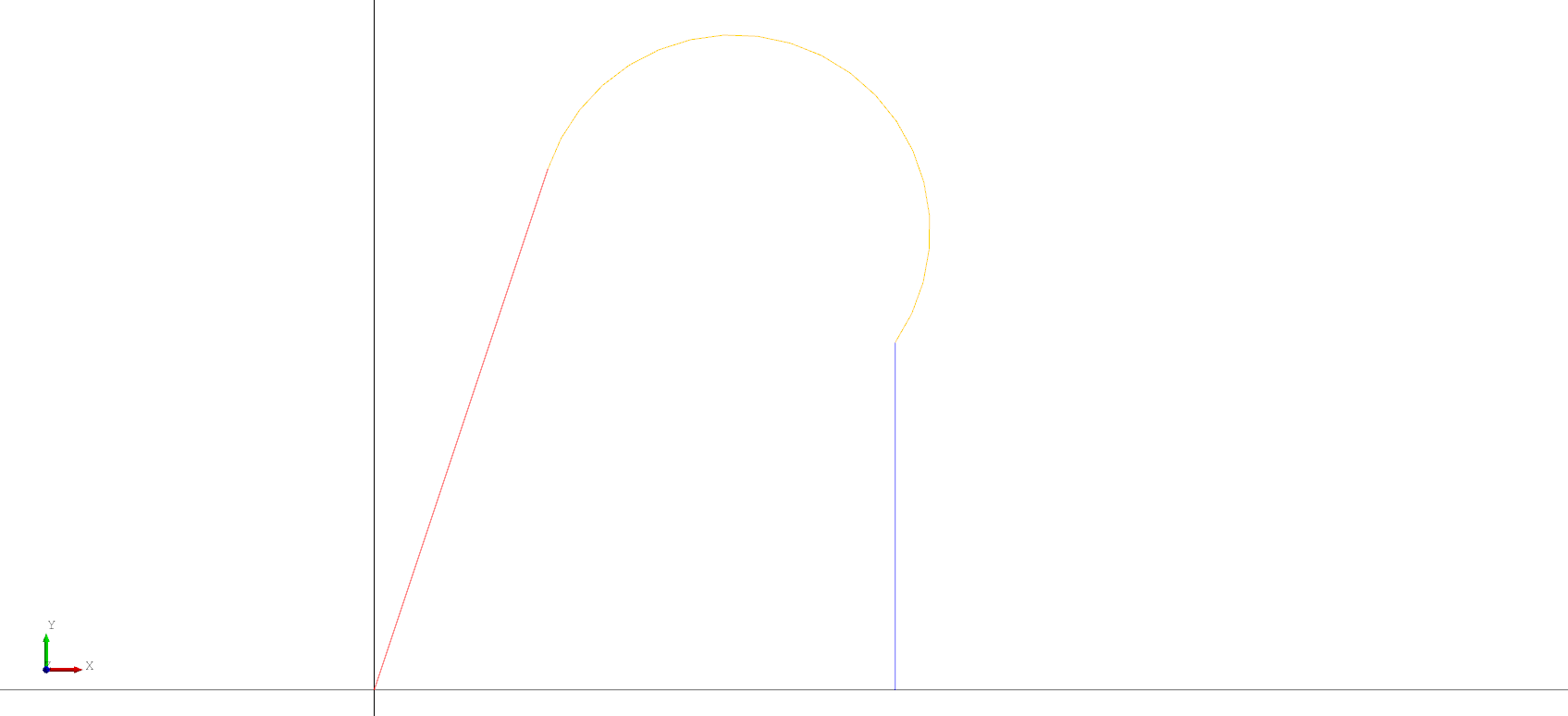## Circles and Ellipse

A circle in the XY plane can be created using the `Circle` declaration. The circle's origin is at the center and is defined by simply setting the `radius`. Similarly an `Ellipse` is defined by specifying a `major_radius` and a `minor_radius`. The `ellipse` can be rotated by setting the `rotation` in radians.

``````# Created in DeclaraCAD

enamldef Assembly(Part):
Circle:
color = 'red'

Ellipse:
color = 'blue'

# For reference
Line: xaxis:
color = 'black'
direction = (1, 0)
Line: yaxis:
color = 'black'
direction = (0, 1)
``````To move it into another plane use a `Transform` or set the `direction` attribute.

## Arcs

An `Arc` can be defined by several different sets of attributes:

• The `position`, `radius` and two angles `alpha1`, and `alpha2` (in radians) (See arc1)
• A `radius` and two points it must pass through (See arc2)
• Three points (See arc3)
• A start point, a start direction, and an end point

Note: When creating an arc using a `position`, `radius`, and two `points` make sure they are all on a single plane.

``````# Created in DeclaraCAD
from math import pi

enamldef Assembly(Part):
Arc: arc1:
color = 'red'
alpha1 = 0
alpha2 = pi/2

Arc: arc2:
color = 'blue'
points = [(0, 3), (3, 0)]

Arc: arc3:
color = 'lime'
points = [arc2.start, (0, 0), arc2.end]

# For reference
Line: xaxis:
color = 'black'
direction = (1, 0)
Line: yaxis:
color = 'black'
direction = (0, 1)
``````The `start` and `end` point of an Arc can be retrieved using the `start` and `end` attributes which is very useful for building wires as we'll see later.

If the second entry in the points list is a direction it is interpreted as the start tangent and the other values are computed.

``````# Created in DeclaraCAD

enamldef Assembly(Part):
Axis:
pass
Polyline: up:
points = [
(0, 0),
(1, 3),
]
Arc:
color = 'orange'
points = [
*up.topology.end_tangent,
down.topology.start_point
]
Polyline: down:
color = 'blue'
points = [
(3, 2),
(3, 0),
]
``````